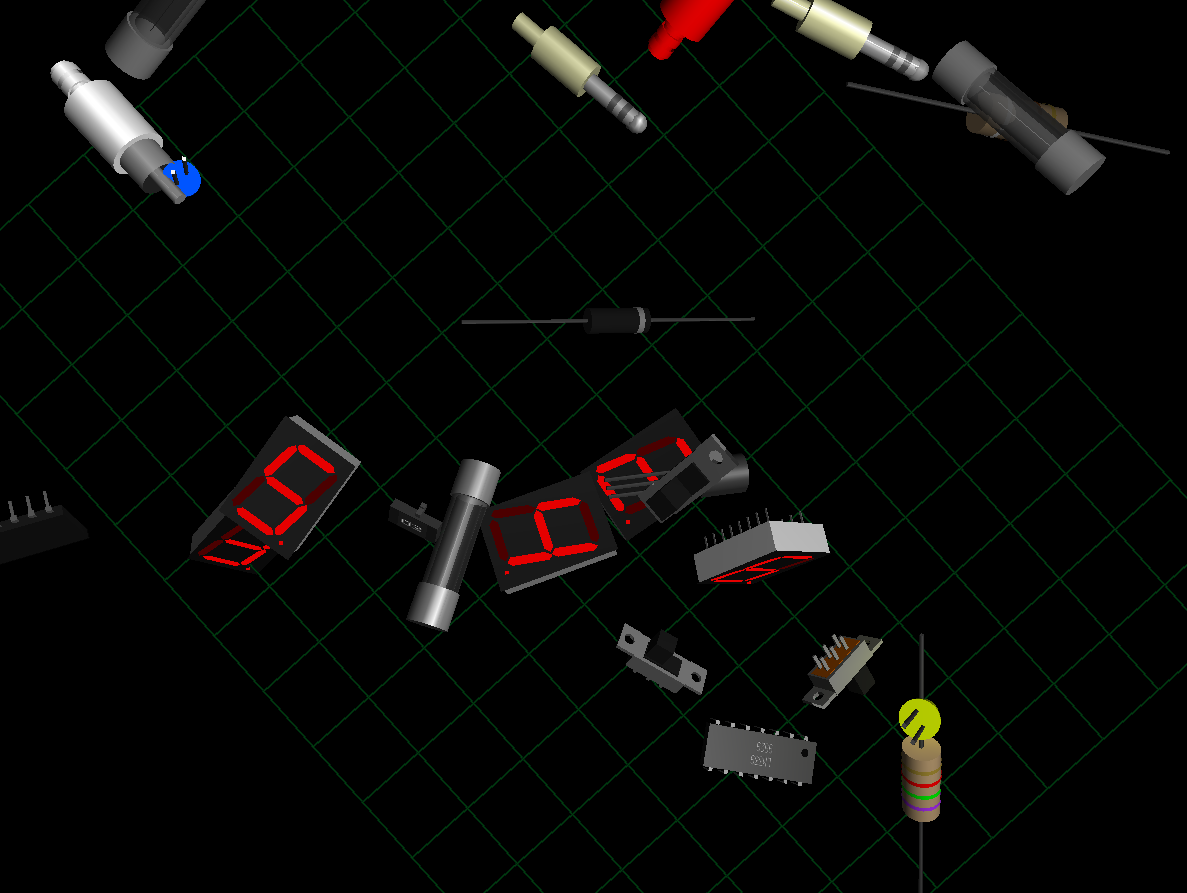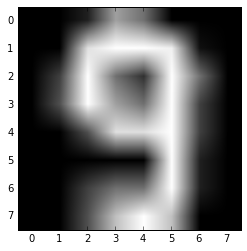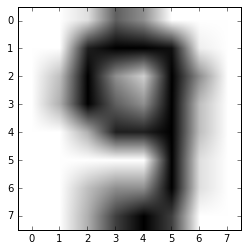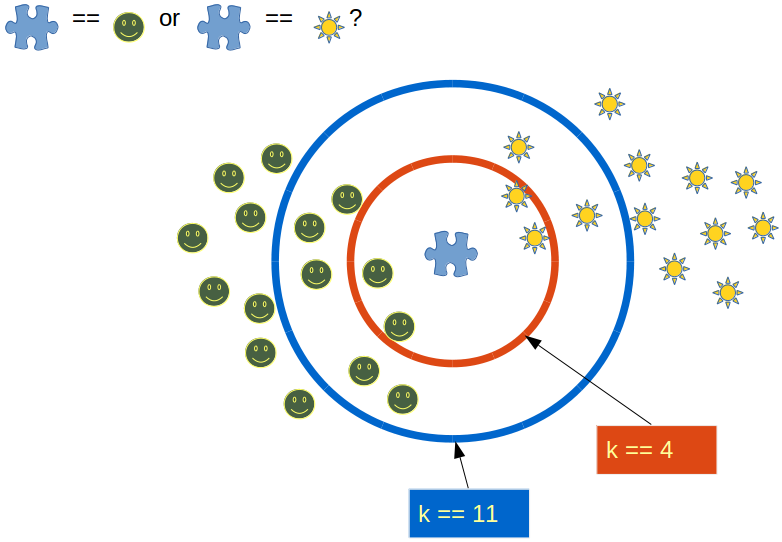# ScikitScikit-learn is a Python module merging classic machine learning algorithms with the world of scientific Python packages (NumPy, SciPy, matplotlib).

## Our Learning Set: "digits"

%matplotlib inline

import numpy as np
from sklearn import datasets
print(type(digits))

<class 'sklearn.datasets.base.Bunch'>


The digits dataset is a dictionary-like objects, containing the actual data and some metadata.

print(digits.data)

[[  0.   0.   5. ...,   0.   0.   0.]
[  0.   0.   0. ...,  10.   0.   0.]
[  0.   0.   0. ...,  16.   9.   0.]
...,
[  0.   0.   1. ...,   6.   0.   0.]
[  0.   0.   2. ...,  12.   0.   0.]
[  0.   0.  10. ...,  12.   1.   0.]]


digits.data contains the features, i.e. images of handwritten images of digits, which can be used for classification.

digits.target

Output: :
array([0, 1, 2, ..., 8, 9, 8])
len(digits.data), len(digits.target)

Output: :
(1797, 1797)

digits.target contain the labels, i.e. digits from 0 to 9 for the digits of digits.data. The data "digits" is a 2 D array with the shape (number of samples, number of features). In our case, a sample is an image of shape (8, 8):

print(digits.target, digits.data)
print(digits.images)

0 [  0.   0.   5.  13.   9.   1.   0.   0.   0.   0.  13.  15.  10.  15.   5.
0.   0.   3.  15.   2.   0.  11.   8.   0.   0.   4.  12.   0.   0.   8.
8.   0.   0.   5.   8.   0.   0.   9.   8.   0.   0.   4.  11.   0.   1.
12.   7.   0.   0.   2.  14.   5.  10.  12.   0.   0.   0.   0.   6.  13.
10.   0.   0.   0.]
[[  0.   0.   5.  13.   9.   1.   0.   0.]
[  0.   0.  13.  15.  10.  15.   5.   0.]
[  0.   3.  15.   2.   0.  11.   8.   0.]
[  0.   4.  12.   0.   0.   8.   8.   0.]
[  0.   5.   8.   0.   0.   9.   8.   0.]
[  0.   4.  11.   0.   1.  12.   7.   0.]
[  0.   2.  14.   5.  10.  12.   0.   0.]
[  0.   0.   6.  13.  10.   0.   0.   0.]]


## Learning and Predicting

We want to predict for a given image, which digit it depicts. Our data set contains samples for the classes 0 (zero) to 9 (nine). We will use these samples to fit an estimator so that we can predict unseen samples as well.

In scikit-learn, an estimator for classification is a Python object that implements the methods fit(X,y) and predict(T).

An example of an estimator is the class sklearn.svm.SVC that implements support vector classification. The constructor of an estimator takes as arguments the parameters of the model, but for the time being, we will consider the estimator as a black box:

from sklearn import svm            # import support vector machine
classifier = svm.SVC(gamma=0.001, C=100.)

classifier.fit(digits.data[:-3], digits.target[:-3])

Output: :
SVC(C=100.0, cache_size=200, class_weight=None, coef0=0.0,
decision_function_shape=None, degree=3, gamma=0.001, kernel='rbf',
max_iter=-1, probability=False, random_state=None, shrinking=True,
tol=0.001, verbose=False)

The classifier, which we have created with svm.SVC, is an estimator object. In general the scikit-learn API provides estimator objects, which can be any object that can learn from data. Learning can be done by classification, regression or clustering algorithm or a transformer that extracts/filters useful features from raw data.

All estimator objects expose a fit method that takes a dataset (usually a 2-d array):

classifier.predict(digits.data[-3:])

Output: :
array([8, 9, 8])
digits.target[-3:]

Output: :
array([8, 9, 8])
digits.data[-3]

Output: :
array([  0.,   0.,   1.,  11.,  15.,   1.,   0.,   0.,   0.,   0.,  13.,
16.,   8.,   2.,   1.,   0.,   0.,   0.,  16.,  15.,  10.,  16.,
5.,   0.,   0.,   0.,   8.,  16.,  16.,   7.,   0.,   0.,   0.,
0.,   9.,  16.,  16.,   4.,   0.,   0.,   0.,   0.,  16.,  14.,
16.,  15.,   0.,   0.,   0.,   0.,  15.,  15.,  15.,  16.,   0.,
0.,   0.,   0.,   2.,   9.,  13.,   6.,   0.,   0.])
import matplotlib.pyplot as plt
from PIL import Image
img = Image.fromarray(np.uint8(digits.images[-2]))

plt.gray()
plt.imshow(img)
plt.show()plt.imshow(digits.images[-2], cmap=plt.cm.gray_r)

Output: :
<matplotlib.image.AxesImage at 0x7f5ef7c42898>## Iris Dataset

The Iris flower data set is a multivariate data set introduced by Ronald Fisher in his 1936 paper "The use of multiple measurements in taxonomic problems as an example of linear discriminant analysis."

The data set consists of 50 samples from each of three species of Iris

• Iris setosa,
• Iris virginica and
• Iris versicolor).

Four features were measured from each sample the length and the width of the sepals and petals, in centimetres.

Based on the combination of these four features, Fisher developed a linear discriminant model to distinguish the species from each other.

## Saving Trained Models

It's possible to keep a trained model persistently with the pickle module.

In the following example, we want to demonstrate how to learn a classifier and save it for later usage with the pickle module of Python:

from sklearn import svm, datasets
import pickle

clf = svm.SVC()

X, y = iris.data, iris.target
clf.fit(X, y)

Output: :
SVC(C=1.0, cache_size=200, class_weight=None, coef0=0.0,
decision_function_shape=None, degree=3, gamma='auto', kernel='rbf',
max_iter=-1, probability=False, random_state=None, shrinking=True,
tol=0.001, verbose=False)
fname = open("classifiers/iris.pkl", "bw")
pickle.dump(clf, fname)

fname = open("classifiers/iris.pkl", "br")

clf2.predict(iris.data[::5])

Output: :
array([0, 0, 0, 0, 0, 0, 0, 0, 0, 0, 1, 1, 1, 1, 1, 1, 1, 1, 1, 1, 2, 2, 2,
2, 2, 2, 2, 2, 2, 2])
iris.target[::5]

Output: :
array([0, 0, 0, 0, 0, 0, 0, 0, 0, 0, 1, 1, 1, 1, 1, 1, 1, 1, 1, 1, 2, 2, 2,
2, 2, 2, 2, 2, 2, 2])

Now, we will do the same with joblib package from sklearn.externals. joblib is more efficient on big data:

from sklearn.externals import joblib
joblib.dump(clf, 'classifiers/iris2.pkl')

clf3.predict(iris.data[::5])

Output: :
array([0, 0, 0, 0, 0, 0, 0, 0, 0, 0, 1, 1, 1, 1, 1, 1, 1, 1, 1, 1, 2, 2, 2,
2, 2, 2, 2, 2, 2, 2])

## Statistical-learning for Scientific Data Processing

We saw that the "iris dataset" consists of 150 observations of irises, i.e. the samples. Each oberservation is described by four features (the length and the width of the sepals and petals).

In general, we can say that Scikit-learn deals with learning information from one or more datasets that are represented as 2D arrays. Such an array can be seen as a list of multi-dimensional observations. The first axis of such an array is the samples axis and the second one is the features axis.

### Supervised Learning

Supervised learning consists in the task of finding or deducing a function from labeled training data. The training data consist of a set of training examples. In other words: We have the actual data X and the corresponding "targets" y, also called "labels". Often y is a one dimensional array.

An estimator in scikit-learn provides a fit method to fit the model: fit(X, y). It also supplies a predict method which returns predicted labels y for (unlabeled) observations X: predict(X) --> y.

## Instance Based Learning -- -k-nearest-neighbor

Instance based learning works directly on the learned samples, instead of creating rules compared to other classification methods.

Way of working: Each new instance is compared with the already existing instances. The instances are compared by using a distance metric. The instance with the closest distance value detwermines the class for the new instance. This classification method is called nearest-neighbor classification.

In [ ]:
### k-nearest-neighbor from Scratch

In [ ]:
import numpy as np
from sklearn import datasets
iris_X = iris.data
iris_y = iris.target
print(iris_X[:8])


We create a learnsetfrom the sets above. We use permutation from np.random to split the data randomly:

np.random.seed(42)
indices = np.random.permutation(len(iris_X))
n_training_samples = 12
iris_X_train = iris_X[indices[:-n_training_samples]]
iris_y_train = iris_y[indices[:-n_training_samples]]
iris_X_test = iris_X[indices[-n_training_samples:]]
iris_y_test = iris_y[indices[-n_training_samples:]]
print(iris_X_test)

[[ 5.7  2.8  4.1  1.3]
[ 6.5  3.   5.5  1.8]
[ 6.3  2.3  4.4  1.3]
[ 6.4  2.9  4.3  1.3]
[ 5.6  2.8  4.9  2. ]
[ 5.9  3.   5.1  1.8]
[ 5.4  3.4  1.7  0.2]
[ 6.1  2.8  4.   1.3]
[ 4.9  2.5  4.5  1.7]
[ 5.8  4.   1.2  0.2]
[ 5.8  2.6  4.   1.2]
[ 7.1  3.   5.9  2.1]]


To determine the similarity between to instances, we need a distance function. In our example, the Euclidean distance is ideal:

def distance(instance1, instance2):
# just in case, if the instances are lists or tuples:
instance1 = np.array(instance1)
instance2 = np.array(instance2)

return np.linalg.norm(instance1 - instance2)

print(distance([4, 3, 2], [1, 1,1]))

3.74165738677

def get_neighbors(training_set, test_instance, k):
distances = []
for training_instance in training_set:
dist = distance(test_instance, training_instance[:-1])
distances.append((training_instance, dist))
distances.sort(key=lambda x: x)
neighbors = []
for i in range(k):
neighbors.append(distances[i])
return neighbors

train_set = [(1, 2, 2, 'apple'),
(-3, -2, 0,  'banana'),
(1, 1, 3, 'apple'),
(-3, -3, -1,  'banana')
]

k = 1
for test_instance in [(0, 0, 0), (2, 2, 2), (-3, -1, 0)]:
neighbors = get_neighbors(train_set, test_instance, 2)
print(test_instance, neighbors)

(0, 0, 0) [(1, 2, 2, 'apple'), (1, 1, 3, 'apple')]
(2, 2, 2) [(1, 2, 2, 'apple'), (1, 1, 3, 'apple')]
(-3, -1, 0) [(-3, -2, 0, 'banana'), (-3, -3, -1, 'banana')]In [ ]: